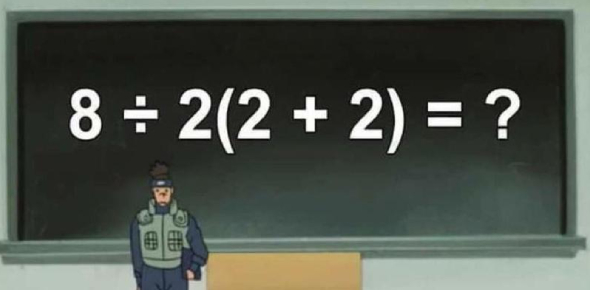# A Basic Math Quiz For 3rd Grade Students

8 Questions | Total Attempts: 19725SettingsThe secret to being good when it comes to math is a constant revision of some problematic areas. If you are in the third grade and are looking for revision material, then the quiz below is perfect for you. Give it a try and see what you have understood so far from math class. All the best!

• 1.
Which of the following is the same as 6 X 8?
• A.

6+6+6+66+6+6

• B.

8+88+88+88+888

• C.

8+8+8+8+8+8

• D.

8-888-888888-88-8

• 2.
What number comes after 8 tens and 8 ones?
• A.

81

• B.

89

• C.

82

• D.

810

• 3.
Some letters in the word below are written using straight lines and some using curved lines.......... CANDLE how many letters are written using only straight lines?
• A.

2

• B.

4

• C.

5

• D.

6

• 4.
How much more than 33 is 55?
• A.

20

• B.

22

• C.

55

• D.

88

• 5.
15+7 is the same as _____ more than 20?
• A.

2

• B.

12

• C.

22

• D.

42

• 6.
Which of the following is the same as 5 times 7?
• A.

5+5+5+5+5

• B.

7+7+7+7+7

• C.

7 x 7 x 7x 7 x7

• D.

5+5+5+5+5

• 7.
Rita has some stamps.....jolly gave her 13 more stamps....if Rita has 29 stamps now, how many did she have in the beginning?
• A.

16

• B.

26

• C.

32

• D.

42

• 8.
Which of these lines say that Rubina has NO balls?
• A.

Rubina has 0 balls

• B.

Rubina has 10 balls

• C.

Rubina has 20 balls

• D.

Rubina has 100 balls

Related TopicsBack to top